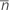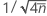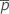# Methods and formulas for P Chart Diagnostic

Select the method or formula of your choice.

## Plotted points

Minitab calculates the adjusted defective counts (ai) as follows:

### Transformed counts

The adjusted counts are then transformed using the following formula:

Then Minitab creates a standard normal probability plot of the transformed counts using the method specified in File > Options > Individual Graphs > Probability Plots.

### Notation

TermDescription
dicount of defectives for subgroup i
nisize of subgroup iaverage subgroup size

## Ratio of observed variation to expected variation

### Expected variation

The expected variation is equal to the standard deviation of the transformed counts (Xi), which is equal to.

### Observed variation

To calculate the observed variation, Minitab calculates normal scores (Y,) for the transformed counts as follows:

where NSCOR is the Normal scores function (available by choosing Calc > Calculator).

For the next step, only the middle 50% of the Xi values are used, along with their corresponding Yi values. Xi values are excluded if they are less than the 25th percentile or greater than the 75th percentile.

Minitab fits a least squares regression model with Yi as the response and Xi as the predictor:

The observed variation is then 1 / β1.

### Ratio

The ratio of observed variation to expected variation is calculated as follows:

### Notation

TermDescriptionaverage subgroup size
β0intercept from the least squares regression equation
β1slope coefficient from the least squares regression equation

## 95% confidence limits for ratio

### upper confidence limit

The upper confidence limit for the ratio is calculated as follows:

whereis the mean proportion of defectives, calculated as follows:

### lower confidence limit

For the lower confidence limit for the ratio, Minitab uses a conservative, fixed value of 60%.

### Notation

TermDescription
mnumber of subgroupsaverage subgroup size
di count of defectives for subgroup i
nisize of subgroup i

## Decision

Minitab compares the ratio of observed variation to expected variation to the 95% upper confidence limit and the 95% lower confidence limit.
If ratio > upper confidence limit
If the ratio is greater than the upper confidence limit, then using a traditional P chart with the data may result in an elevated false alarm rate. In this case, a Laney P' chart is recommended.
If ratio < lower confidence limit
If the ratio is less than the lower confidence limit, then using a traditional P chart with the data may result in control limits that are too wide. In this case, a Laney P' chart is recommended.
By using this site you agree to the use of cookies for analytics and personalized content.  Read our policy